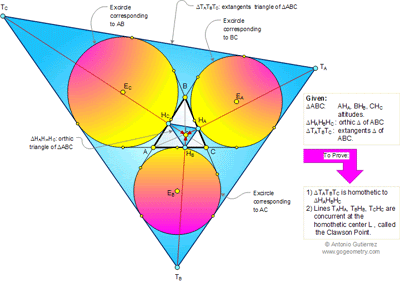## Thursday, June 30, 2011

### Problem 630: Clawson Point: Orthic Triangle, Extangents Triangle, Homothecy

Click the figure below to view the Clawson Point.Zoom at: Clawson Point
Level: High School, SAT Prep, College geometry

1.http://img69.imageshack.us/img69/8631/problem630.png

1. Let BC cut HcHb at N and 2 tangents (to circles Es and Eb) TcTb and BC cut EcEs at M
Note that EcEs is angle bisector of angle (TcMB) and quadrilateral BHcHbC is cyclic.
angle (CHcHb)= angle (CBHb)=90-C
In triangle BHcN we have y= 180-B-(90+90-C)= C-B
In triangle AHbEs we have angle (HbAEs)=90-A/2
In triangle BAM we have x=180-B-A-(90-A/2)=90-B-A/2
2x=180-2B-A= C-B
So y=2x and TaTc // HbHc . Similarly we also have TbTa//HbHa and TaTc//HaHc
And triangle TaTbTc similar to Tri. HaHbHc with corresponding sides parallel

2. Let TcHc cut TbHb at L. we will prove that L, Ha and Ta are collinear
Tri. LHcHb similar to tri. LTcTa ( case AA)
So HcHb/TcTb=LHb/LTb=HbHa/TaTb
Tri. LHbHa similar to Tri. LTbTa ( case SAS)
So angle (HbLHa)= angle (TbLTa) and L, Ha and Ta are collinear

And lines TaHa, TbHb, TcHc are concurrent at L

Peter Tran

2.below is an improved solution in comparing with my previous solution:
http://img839.imageshack.us/img839/2746/problem6301.png
1. Let AC cut TcTb at P
Note that TaTc, BC, AB, AC are the tangents of circles Ec and Eb so EcEb is the axis of symmetry
So angle(APTb)= angle(ABC)
Quadrilateral BHcHbC is cyclic so angle(HcHbA)= angle(HcBC) …both angles supplement to angle(HcHbC)
And Angle(APTb)=angle(HcHbA)=angle(ABC) so TcTb//HcHb
similarly we will have TaTc//HaHc and TaTb//HaHb
And triangle TaTbTc similar to Tri. HaHbHc with corresponding sides parallel

2. Let TcHc cut TbHb at L. we will prove that L, Ha and Ta are collinear
Tri. LHcHb similar to tri. LTcTa ( case AA)
So HcHb/TcTb=LHb/LTb=HbHa/TaTb
Tri. LHbHa similar to Tri. LTbTa ( case SAS)
So angle (HbLHa)= angle (TbLTa) and L, Ha and Ta are collinear

And lines TaHa, TbHb, TcHc are concurrent at L

Peter Tran

3.it was my favorite subject in highschool. What level is it? University at least, by all the calculations.

4.